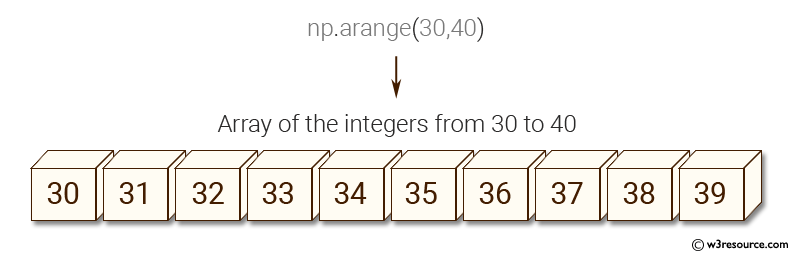﻿ NumPy: Create an array of the integers from 30 to 70 - w3resource# NumPy: Create an array of the integers from 30 to 70

## NumPy: Basic Exercise-14 with Solution

Write a NumPy program to create an array of the integers from 30 to 70.

Sample Solution :

Python Code :

``````import numpy as np
array=np.arange(30,71)
print("Array of the integers from 30 to70")
print(array)
``````

Sample Output:

```Array of the integers from 30 to70
[30 31 32 33 34 35 36 37 38 39 40 41 42 43 44 45 46 47 48 49 50 51 52 53 54
55 56 57 58 59 60 61 62 63 64 65 66 67 68 69 70]
```

Pictorial Presentation:Python Code Editor:

Have another way to solve this solution? Contribute your code (and comments) through Disqus.

What is the difficulty level of this exercise?

Test your Python skills with w3resource's quiz

﻿

## Python: Tips of the Day

Checks if the given number falls within the given range.

Example:

```def tips_range(n, start, end = 0):
return start <= n <= end if end >= start else end <= n <= start
print(tips_range(2, 4, 6))
print(tips_range(4, 8))
print(tips_range(1, 3, 5))
print(tips_range(1, 3))
```

Output:

```False
True
False
True
```